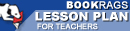Lesson Plans

# Journey Through Genius: The Great Theorems of Mathematics Quiz | Eight Week Quiz B

This set of Lesson Plans consists of approximately 142 pages of tests, essay questions, lessons, and other teaching materials.
 View a FREE sampleName: _________________________ Period: ___________________

This quiz consists of 5 multiple choice and 5 short answer questions through Euclid and the Infinitude of Primes.

## Multiple Choice Questions

1. In general, what did Euclid's number theory describe?
(a) The nature of measuring geometry.
(b) The relationship of fractions to decimals.
(c) The relationship of decimals to integers.
(d) The nature of whole numbers.

2. That properties of specific shapes were early Egyptians aware of?
(a) Right triangles.
(b) Irregular solids.
(c) Parallelograms.
(d) Pi and the diameter of a circle.

3. What did Ferdinand Lindeman prove in 1882?
(a) It is impossible to find the square of a semicircle.
(b) That the square root of the hypotenuse of a right triangle can not be found.
(c) It is possible to find the square of a circle.
(d) That the square of a circle can not be found with a compass and a straight-edge.

4. What is the name for determining the area of an enclosed space by constructing a square of equivalent area?
(a) Cubation.
(b) Square root.
(c) Quadrature.
(d) Triangulation.

5. Which of the following was one of Euclid's great theorems?
(a) There exists an finite number of prime numbers.
(b) There exists only infinite and whole numbers.
(c) Prime numbers are more comples than discrete numbers.
(d) There exists an infinite number of prime numbers.

## Short Answer Questions

1. Which of the following were an example of twin primes?

2. Numbers whose divisor add up to itself, was considered which type of number according to Euclid?

3. Which of the following was NOT one of the things Dunham claimed was ingenious about Euclid's proof of the Pythagorean theorem?

4. How many definitions were stated in Elements?

5. What was Hippocrates's great advance to mathematics?

(see the answer key)

 This section contains 339 words (approx. 2 pages at 300 words per page) View a FREE sampleCopyrightsJourney Through Genius: The Great Theorems of Mathematics from BookRags. (c)2019 BookRags, Inc. All rights reserved.
Follow Us on Facebook